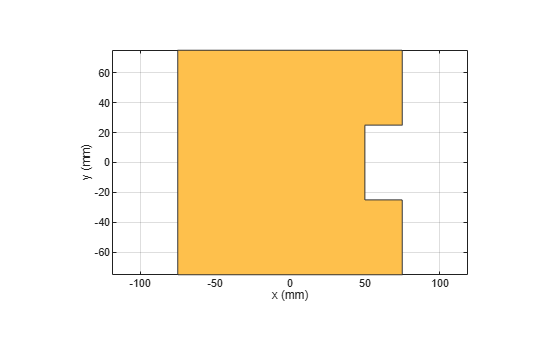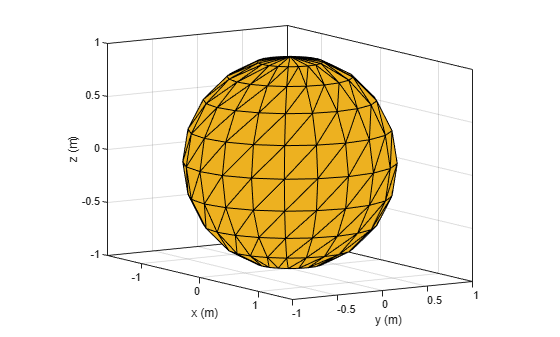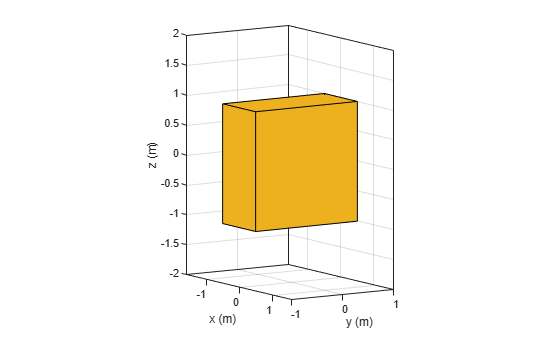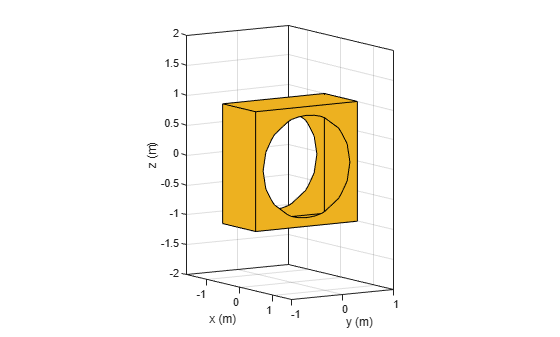# subtract

Boolean subtraction operation on two shapes

## Syntax

``subtract(shape1,shape2)``
``subtract(shape1,shape2,RetainShape=0)``
``c = subtract(shape1,shape2)``
``c = subtract(shape1,shape2,RetainShape=0)``

## Description

example

````subtract(shape1,shape2)` carves out the intersecting region of the `shape2` and `shape1` from `shape1` using the subtract operation and plots the resultant shape retaining the enclosed region of `shape2` within `shape1`. Adjust the `Transparency` property of the shapes to view the retained individual shape portion in the resultant shape. Alternatively, you can also use the `'-'` operator to carve out a shape from other shape (`shape1 - shape2`).```
````subtract(shape1,shape2,RetainShape=0)` carves out the intersecting region of the `shape2` and `shape1` from `shape1` and plots the resultant shape while merging both shapes.```

example

````c = subtract(shape1,shape2)` carves out the intersecting region of the `shape2` and `shape1` from `shape1` using the subtract operation retaining the enclosed region of `shape2` within `shape1` and returns a polygon object for resultant 2-D shape or a custom 3-D object for resultant 3-D shape. Adjust the `Transparency` property of the shapes to view the retained individual shape portion in the resultant shape. Alternatively, you can also use the `'-'` operator to perform the subtraction operation (```c = shape1 - shape2```).```
````c = subtract(shape1,shape2,RetainShape=0)` carves out the intersecting region of the `shape2` and `shape1` from `shape1` while merging both the shapes and returns a custom 3-D object for the resultant 3-D shape.```

## Examples

collapse all

Create a rectangle with a length of 0.15 m, and a width of 0.15 m.

`r = antenna.Rectangle(Length=0.15,Width=0.15);`

Create a second rectangle with a length of 0.05 m, and a width of 0.05 m. Set the center of the second rectangle at half the length of the first rectangle r.

`n = antenna.Rectangle(Center=[0.075,0],Length=0.05,Width=0.05);`

Create and view a notched rectangle by subtracting n from r.

```rn = r-n; show(rn)```Calculate the area of the notched rectangle.

`area(rn)`
```ans = 0.0212 ```

This example shows how to create a hollow sqaure nut shape by carving out a sphere from a box shape.

Create and view a spherical shape.

```a = shape.Sphere(Color=[0.9290 0.6940 0.1250]); show(a)```Create and view a box shape.

```b = shape.Box(Color=[0.9290 0.6940 0.1250]); show(b)```Carve out the sphere from the box and view the resultant shape.

`subtract(b,a);`## Input Arguments

collapse all

2-D and 3-D geometric shapes created using shape objects in Custom 2-D and 3-D Antenna.

Example: `antenna.Rectangle,antenna.Circle`

Example: `shape.Box,shape.Sphere`

## Output Arguments

collapse all

2-D or 3-D shape obtained after carving out `shape2` from `shape1`.

Example: `antenna.Polygon`

Example: `shape.Polygon`

Example: `shape.Custom3D`

## Version History

Introduced in R2017a